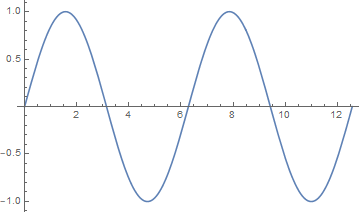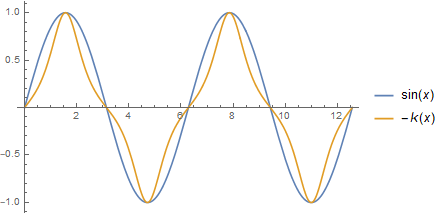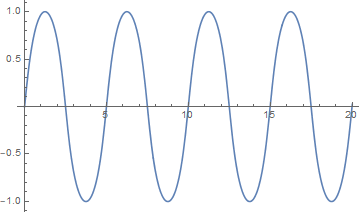# Self-curvature

If you look at a sine wave, its curvature is sorta like its values. When sine is zero, the curve is essentially a straight line, and the curvature is zero. When sine is at its maximum, the function bends the most and so the curvature is at a maximum.This makes sense analytically. The second derivative is something like curvature, and the second derivative of sin(x) is -sin(x). The negative sign suggests that if we look at signed curvature rather than absolute curvature, then the values of a sine curve are roughly proportional to the negative of the curvature at each point.

Here’s a plot showing sin(x) and its negative curvature.Steven Wilkinson wrote an article  addressing the question of whether there is a curve so that the curvature at each point is proportional to the value of the curve. The curvature of a graph of y = y(x) isand so a curve that is proportional to its curvature is a solution to the nonlinear differential equationGeneral theory says that there should at least be a solution in a (possibly small) neighborhood of 0, but it’s not obvious a priori that interesting solutions exist. It turns out that if we pick the proportionality constant a = -1 and the initial conditions y(0) = 0 and y ‘(0) = √3 then we get a periodic solution that looks something like a sine.

The following Mathematica code will plot the function for us.

    s = NDSolve[
{y''[x] = -y[x] (1 + y'[x]^2)^(-3/2),
y == 0, y' == Sqrt},
y, {x, 0, 10}]

Plot[Evaluate[{y[x] /. s}], {x, 0, 10}]


The plot looks a lot like a sine wave. In fact, I imagine that when asked to draw a sine wave, most people would draw something that looks more like this than like an actual sine wave.## Related posts

 Steven Wilkison. Self-Curvature Curves. Mathematics Magazine, Vol. 82, No. 5 (December 2009), pp. 354-359

## 4 thoughts on “Self-curvature”

1. Jim Shapiro

Shouldn’t the exponent be (3 / 2), instead of (-3 / 2).

2. Thanks! I thought that didn’t look right. :)

3. Andrew

Are those zero crossings exactly at multiples of 5, or something else?

4. Almost. The exact value is given by an integral in the paper. To four decimal place the period is 4.9967.